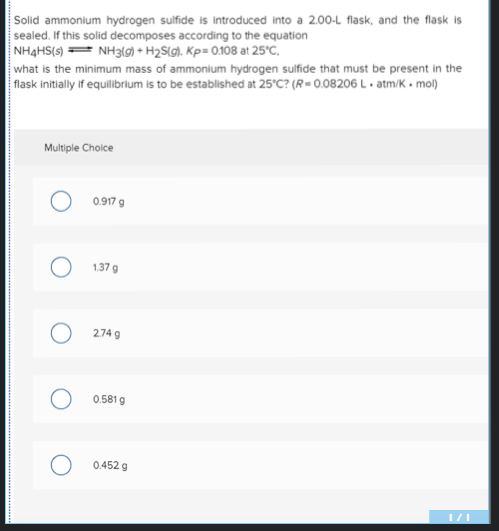# Solid ammonium hydrogen sulfide is introduced into a 2.00-L flask, and the flask is sealed. If this solid decomposes according to the equation NH4HS(s) NH3(g) + H2S(g), Kp= 0.108 at 25°C, what is the minimum mass of ammonium hydrogen sulfide that must be present in the flask initially if equilibrium is to be established at 25°C? (R= 0.08206 L • atm/K • mol)